Home

# Squared Chromatic Number Without Claws or Large Cliques

## Abstract

Let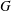$G$ be a claw-free graph on$n$ vertices with clique number$\unicode[STIX]{x1D714}$ , and consider the chromatic number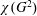$\unicode[STIX]{x1D712}(G^{2})$ of the square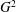$G^{2}$ of$G$ . Writing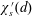$\unicode[STIX]{x1D712}_{s}^{\prime }(d)$ for the supremum of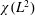$\unicode[STIX]{x1D712}(L^{2})$ over the line graphs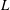$L$ of simple graphs of maximum degree at most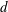$d$ , we prove that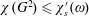$\unicode[STIX]{x1D712}(G^{2})\leqslant \unicode[STIX]{x1D712}_{s}^{\prime }(\unicode[STIX]{x1D714})$ for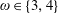$\unicode[STIX]{x1D714}\in \{3,4\}$ . For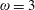$\unicode[STIX]{x1D714}=3$ , this implies the sharp bound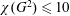$\unicode[STIX]{x1D712}(G^{2})\leqslant 10$ . For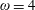$\unicode[STIX]{x1D714}=4$ , this implies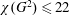$\unicode[STIX]{x1D712}(G^{2})\leqslant 22$ , which is within 2 of the conjectured best bound. This work is motivated by a strengthened form of a conjecture of Erdős and Nešetřil.

## Footnotes

Hide All

Author W. C. v. B. was supported by NWO grant 613.001.217. Author R. J. K. is supported by a NWO Vidi Grant, reference 639.032.614.

## References

Hide All
MathJax
MathJax is a JavaScript display engine for mathematics. For more information see http://www.mathjax.org.

# Squared Chromatic Number Without Claws or Large Cliques

## Metrics

### Full text viewsFull text views reflects the number of PDF downloads, PDFs sent to Google Drive, Dropbox and Kindle and HTML full text views.

Total number of HTML views: 0
Total number of PDF views: 0 *Loading metrics...

### Abstract viewsAbstract views reflect the number of visits to the article landing page.

Total abstract views: 0 *Loading metrics...

* Views captured on Cambridge Core between <date>. This data will be updated every 24 hours.

Usage data cannot currently be displayed